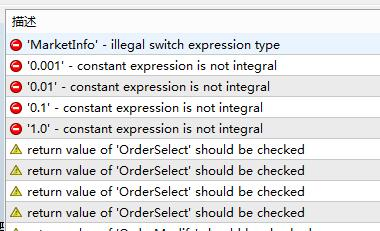# 'MarketInfo' - illegal switch expression type 这段代码没错啊MQ4怎么过不了啊```int init() {
if (Digits == 2 || Digits == 4) gi_420 = 1;
else gi_420 = 10;
gi_416 = AccountNumber();
gd_280 = MarketInfo(Symbol(), MODE_SPREAD) * Point * gi_420;
switch (MarketInfo(Symbol(), MODE_MINLOT)) {
case 0.001:
gd_204 = 3;
break;
case 0.01:
gd_204 = 2;
break;
case 0.1:
gd_204 = 1;
break;
case 1.0:
gd_204 = 0;
}
if (SafeEquityStopOut) gs_off_372 = "ON";
if (IsDemo()) gs_live_380 = "DEMO";
if (Period() != PERIOD_M15) {
Print("FGT ERROR ::无效的期限，请切换到M1.");
gs_396 = "无效的期限，请切换到M1";
gi_408 = FALSE;
}
return (0);
}

```

peng wu:

eajq.PNG  73 kb